# NCERT Solutions for Class 9 Maths Chapter 9 Areas of Parallelograms and Triangles| PDF Download

Chapter 9 Areas of Parallelograms and Triangles Class 9 Maths NCERT Solutions is available on this page that will help you in completing your homework on time and obtain maximum marks in the exams. You can also Download PDF of Chapter 9 Areas of Parallelograms and Triangles NCERT Solutions Class 9 Maths to practice in a better manner. There are variety of concepts given in this chapter 6 Class 9 Maths textbook that will develop your necessary skill to solve more and more questions.

These NCERT Solutions for Class 9 will prove useful guide in making a student confident. These solutions are updated according to the latest NCERT Class 9 Maths textbook. Chapter 9 NCERT Solutions will develop you understanding of the chapter and make a student confident.Page No: 155

Exercise 9.1

1. Which of the following figures lie on the same base and between the same parallels. In such a case, write the common base and the two parallels.(i) Trapezium ABCD and ΔPDC lie on the same DC and between the same parallel lines AB and DC.
(ii) Parallelogram PQRS and  trapezium SMNR lie on the same base SR but not between the same parallel lines.
(iii) Parallelogram PQRS and ΔRTQ lie on the same base QR and between the same parallel lines QR and PS.
(iv) Parallelogram ABCD and ΔPQR do not lie on the same base but between the same parallel lines BC and AD.
(v) Quadrilateral ABQD and trapezium APCD lie on the same base AD and between the same parallel lines AD and BQ.
(vi) Parallelogram PQRS and parallelogram ABCD do not lie on the same base SR but between the same parallel lines SR and PQ.

Page No: 159

Exercise 9.2

1. In Fig. 9.15, ABCD is a parallelogram, AE ⊥ DC and CF ⊥ AD. If AB = 16 cm, AE = 8 cm and CF = 10 cm, find AD.Given,
AB = CD = 16 cm (Opposite sides of a parallelogram)
CF = 10 cm and AE = 8 cm
Now,
Area of parallelogram = Base × Altitude
= CD × AE = AD × CF
⇒ 16 × 8 = AD × 10

2. If E,F,G and H are respectively the mid-points of the sides of a parallelogram ABCD, show that
ar (EFGH) = 1/2 ar(ABCD).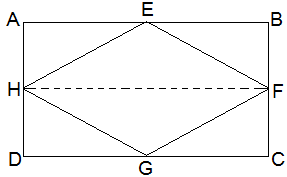Given,
E,F,G and H are respectively the mid-points of the sides of a parallelogram ABCD.
To Prove,
ar (EFGH) = 1/2 ar(ABCD)
Construction,
H and F are joined.
Proof,
AD || BC and AD = BC (Opposite sides of a parallelogram)
⇒ 1/2 AD = 1/2 BC
Also,
AH || BF and and DH || CF
⇒ AH = BF and DH = CF (H and F are mid points)
Thus, ABFH and HFCD are parallelograms.
Now,
ΔEFH and ||gm ABFH lie on the same base FH and between the same parallel lines AB and HF.
∴ area of EFH = 1/2 area of ABFH --- (i)
also, area of GHF = 1/2 area of HFCD --- (ii)
area of ΔEFH + area of ΔGHF = 1/2 area of ABFH + 1/2 area of HFCD
⇒ area of EFGH = area of ABFH
⇒ ar (EFGH) = 1/2 ar(ABCD)

3. P and Q are any two points lying on the sides DC and AD respectively of a parallelogram ABCD. Show that ar(APB) = ar(BQC).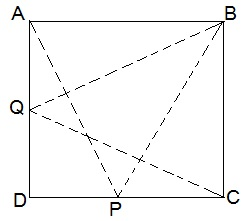ΔAPB and ||gm ABCD are on the same base AB and between same parallel AB and DC.
Therefore,
ar(ΔAPB) = 1/2 ar(||gm ABCD) --- (i)
Similarly,
ar(ΔBQC) = 1/2 ar(||gm ABCD) --- (ii)
From (i) and (ii),
we have ar(ΔAPB) = ar(ΔBQC)

4. In Fig. 9.16, P is a point in the interior of a parallelogram ABCD. Show that
(i) ar(APB) + ar(PCD) = 1/2 ar(ABCD)
(ii) ar(APD) + ar(PBC) = ar(APB) + ar(PCD)
[Hint : Through P, draw a line parallel to AB.](i) A line GH is drawn parallel to AB passing through P.
In a parallelogram,
AB || GH (by construction) --- (i)
Thus,
AD || BC ⇒ AG || BH --- (ii)
From equations (i) and (ii),
ABHG is a parallelogram.
Now,
In ΔAPB and parallelogram ABHG are lying on the same base AB and between the same parallel lines AB and GH.
∴ ar(ΔAPB) = 1/2 ar(ABHG) --- (iii)
also,
In ΔPCD and parallelogram CDGH are lying on the same base CD and between the same parallel lines CD and GH.
∴ ar(ΔPCD) = 1/2 ar(CDGH) --- (iv)
ar(ΔAPB) + ar(ΔPCD) = 1/2 {ar(ABHG) + ar(CDGH)}
⇒ ar(APB) + ar(PCD) = 1/2 ar(ABCD)

(ii) A line EF is drawn parallel to AD passing through P.
In a parallelogram,
AD || EF (by construction) --- (i)
Thus,
AB || CD ⇒ AE || DF --- (ii)
From equations (i) and (ii),
AEDF is a parallelogram.
Now,
In ΔAPD and parallelogram AEFD are lying on the same base AD and between the same parallel lines AD and EF.
∴ ar(ΔAPD) = 1/2 ar(AEFD) --- (iii)
also,
In ΔPBC and parallelogram BCFE are lying on the same base BC and between the same parallel lines BC and EF.
∴ ar(ΔPBC) = 1/2 ar(BCFE) --- (iv)
ar(ΔAPD) + ar(ΔPBC) = 1/2 {ar(AEFD) + ar(BCFE)}
⇒ ar(APD) + ar(PBC) = ar(APB) + ar(PCD)

5. In Fig. 9.17, PQRS and ABRS are parallelograms and X is any point on side BR. Show that
(i) ar (PQRS) = ar (ABRS)
(ii) ar (AXS) = 1/2 ar (PQRS)(i) Parallelogram PQRS and ABRS lie on the same base SR and between the same parallel lines SR and PB.
∴ ar(PQRS) = ar(ABRS) --- (i)
(ii) In ΔAXS and parallelogram ABRS are lying on the same base AS and between the same parallel lines AS and BR.
∴ ar(ΔAXS) = 1/2 ar(ABRS) --- (ii)
From (i) and (ii),
ar(ΔAXS) = 1/2 ar(PQRS)

Page No: 106

6. A farmer was having a field in the form of a parallelogram PQRS. She took any point A on RS and joined it to points P and Q. In how many parts the fields is divided? What are the shapes of these parts? The farmer wants to sow wheat and pulses in equal portions of the field separately. How should she do it?The field is divided into three parts. The three parts are in the shape of triangle. ΔPSA, ΔPAQ and ΔQAR.
Area of ΔPSA + ΔPAQ + ΔQAR = Area of PQRS --- (i)
Area of ΔPAQ = 1/2 area of PQRS --- (ii)
Triangle and parallelogram on the same base and between the same parallel lines.
From (i) and (ii),
Area of ΔPSA + Area of ΔQAR = 1/2 area of PQRS --- (iii)
Clearly from (ii) and (iii),
Farmer must sow wheat or pulses in ΔPAQ or either in both ΔPSA and ΔQAR.

Page No: 162

Exercise 9.3

1. In Fig.9.23, E is any point on median AD of a ΔABC. Show that ar (ABE) = ar(ACE).Given,
AD is median of ΔABC. Thus, it will divide ΔABC into two triangles of equal area.
∴ ar(ABD) = ar(ACD) --- (i)
also,
ED is the median of ΔABC.
∴ ar(EBD) = ar(ECD) --- (ii)
Subtracting (ii) from (i),
ar(ABD) - ar(EBD) = ar(ACD) - ar(ECD)
⇒ ar(ABE) = ar(ACE)

2. In a triangle ABC, E is the mid-point of median AD. Show that ar(BED) = 1/4 ar(ABC).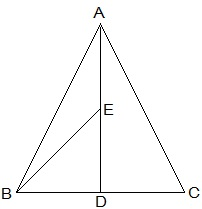ar(BED) = (1/2) × BD × DE
As E is the mid-point of AD,
Thus, AE = DE
As AD is the median on side BC of triangle ABC,
Thus, BD = DC
Therefore,
BD = (1/2)BC --- (ii)
From (i) and (ii),
ar(BED) = (1/2) × (1/2) BC × (1/2)AD
⇒ ar(BED) = (1/2) × (1/2) ar(ABC)
⇒ ar(BED) = 1/4 ar(ABC)

3. Show that the diagonals of a parallelogram divide it into four triangles of equal area.O is the mid point of AC and BD. (diagonals of bisect each other)
In ΔABC, BO is the median.
∴ ar(AOB) = ar(BOC) --- (i)
also,
In ΔBCD, CO is the median.
∴ ar(BOC) = ar(COD) --- (ii)
In ΔACD, OD is the median.
∴ ar(AOD) = ar(COD) --- (iii)
In ΔABD, AO is the median.
∴ ar(AOD) = ar(AOB) --- (iv)
From equations (i), (ii), (iii) and (iv),
ar(BOC) = ar(COD) = ar(AOD) = ar(AOB)
So, the diagonals of a parallelogram divide it into four triangles of equal area.

4. In Fig. 9.24, ABC and ABD are two triangles on the same base AB. If line- segment CD is bisected by AB at O, show that:
ar(ABC) = ar(ABD).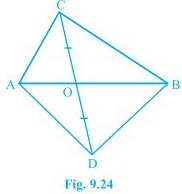In ΔABC,
AO is the median. (CD is bisected by AB at O)
∴ ar(AOC) = ar(AOD) --- (i)
also,
In ΔBCD,
BO is the median. (CD is bisected by AB at O)
∴ ar(BOC) = ar(BOD) --- (ii)
Adding (i) and (ii) we get,
ar(AOC) + ar(BOC) = ar(AOD) + ar(BOD)
⇒ ar(ABC) = ar(ABD)

Page No: 163

5. D, E and F are respectively the mid-points of the sides BC, CA and AB of a ΔABC.
Show that
(i) BDEF is a parallelogram.         (ii) ar(DEF) = 1/4 ar(ABC)
(iii) ar (BDEF) = 1/2 ar(ABC)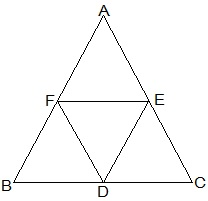(i) In ΔABC,
EF || BC and EF = 1/2 BC (by mid point theorem)
also,
BD = 1/2 BC (D is the mid point)
So, BD = EF
also,
BF and DE will also parallel and equal to each other.
Thus, the pair opposite sides are equal in length and parallel to each other.
∴ BDEF is a parallelogram.

(ii) Proceeding from the result of (i),
BDEF, DCEF, AFDE are parallelograms.
Diagonal of a parallelogram divides it into two triangles of equal area.
∴ ar(ΔBFD) = ar(ΔDEF) (For parallelogram BDEF) --- (i)
also,
ar(ΔAFE) = ar(ΔDEF) (For parallelogram DCEF) --- (ii)
ar(ΔCDE) = ar(ΔDEF) (For parallelogram AFDE) --- (iii)
From (i), (ii) and (iii)
ar(ΔBFD) = ar(ΔAFE) = ar(ΔCDE) = ar(ΔDEF)
⇒ ar(ΔBFD) + ar(ΔAFE) + ar(ΔCDE) + ar(ΔDEF) = arar(ΔABC)
⇒ 4 ar(ΔDEF) = ar(ΔABC)
⇒ ar(DEF) = 1/4 ar(ABC)

(iii) Area (parallelogram BDEF) = ar(ΔDEF) + ar(ΔBDE)
⇒ ar(parallelogram BDEF) = ar(ΔDEF) + ar(ΔDEF)
⇒ ar(parallelogram BDEF) = 2× ar(ΔDEF) ⇒ ar(parallelogram BDEF) = 2× 1/4  ar(ΔABC) ⇒ ar(parallelogram BDEF) = 1/2 ar(ΔABC)

6. In Fig. 9.25, diagonals AC and BD of quadrilateral ABCD intersect at O such that OB = OD.
If AB = CD, then show that:
(i) ar (DOC) = ar (AOB)
(ii) ar (DCB) = ar (ACB)
(iii) DA || CB or ABCD is a parallelogram.
[Hint : From D and B, draw perpendiculars to AC.]Given,
OB = OD and AB = CD
Construction,
DE ⊥ AC and BF ⊥ AC are drawn.
Proof:
(i) In ΔDOE and ΔBOF,
∠DEO = ∠BFO (Perpendiculars)
∠DOE = ∠BOF (Vertically opposite angles)
OD = OB (Given)
Therefore, ΔDOE ≅ ΔBOF by AAS congruence condition.
Thus, DE = BF (By CPCT) --- (i)
also, ar(ΔDOE) = ar(ΔBOF) (Congruent triangles) --- (ii)
Now,
In ΔDEC and ΔBFA,
∠DEC = ∠BFA (Perpendiculars)
CD = AB (Given)
DE = BF (From i)
Therefore,ΔDEC ≅ ΔBFA by RHS congruence condition.
Thus, ar(ΔDEC) = ar(ΔBFA) (Congruent triangles) --- (iii)
ar(ΔDOE) + ar(ΔDEC) = ar(ΔBOF) + ar(ΔBFA)
⇒ ar (DOC) = ar (AOB)

(ii)  ar(ΔDOC) = ar(ΔAOB)
ar(ΔDOC) + ar(ΔOCB) = ar(ΔAOB) + ar(ΔOCB)    (Adding ar(ΔOCB) to both sides)  ⇒ ar(ΔDCB) = ar(ΔACB)   (iii)  ar(ΔDCB) = ar(ΔACB) If two triangles are having same base and equal areas, these will be between same parallels DA || BC --- (iv) For quadrilateral ABCD, one pair of opposite sides are equal (AB = CD) and other pair of opposite sides are parallel. Therefore, ABCD is parallelogram.

7. D and E are points on sides AB and AC respectively of  ΔABC such that ar(DBC) = ar(EBC). Prove that DE || BC.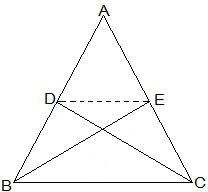ΔDBC and ΔEBC are on the same base BC and also having equal areas. Therefore, they will lie between the same parallel lines. Thus, DE || BC.

8. XY is a line parallel to side BC of a triangle ABC. If BE || AC and CF || AB meet XY at E and F respectively, show that
ar(ΔABE) = ar(ΔAC)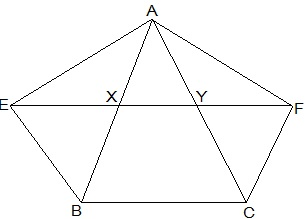Given,
XY || BC, BE || AC and CF || AB
To show,
ar(ΔABE) = ar(ΔAC)
Proof:
EY || BC (XY || BC) --- (i)
also,
BE∥ CY (BE || AC) --- (ii)
From (i) and (ii),
BEYC is a parallelogram. (Both the pairs of opposite sides are parallel.)
Similarly,
BXFC is a parallelogram.
Parallelograms on the same base BC and between the same parallels EF and BC.
ar(BEYC) = ar(BXFC) (Parallelograms on the same base BC and between the same parallels EF and BC) --- (iii)
Also,
△AEB and parallelogram BEYC are on the same base BE and between the same parallels BE and AC.
ar(△AEB) = 1/2ar(BEYC) --- (iv)
Similarly,
△ACF and parallelogram BXFC on the same base CF and between the same parallels CF and AB.
⇒ ar(△ ACF) = 1/2ar(BXFC) --- (v)
From (iii), (iv) and (v),
ar(△AEB) = ar(△ACF)

9. The side AB of a parallelogram ABCD is produced to any point P. A line through A and parallel to CP meets CB produced at Q and then parallelogram PBQR is completed (see Fig. 9.26). Show that
ar(ABCD) = ar(PBQR).
[Hint : Join AC and PQ. Now compare ar(ACQ) and ar(APQ).]AC and PQ are joined.
ar(△ACQ) = ar(△APQ) (On the same base AQ and between the same parallel lines AQ and CP)
⇒ ar(△ACQ) - ar(△ABQ) = ar(△APQ) - ar(△ABQ)
⇒ ar(△ABC) = ar(△QBP) --- (i)
AC and QP are diagonals ABCD and PBQR. Thus,
ar(ABC) = 1/2 ar(ABCD) --- (ii)
ar(QBP) = 1/2 ar(PBQR) --- (iii)
From (ii) and (ii),
1/2 ar(ABCD) = 1/2 ar(PBQR)
⇒ ar(ABCD) = ar(PBQR)

10. Diagonals AC and BD of a trapezium ABCD with AB || DC intersect each other at O. Prove that ar (AOD) = ar (BOC).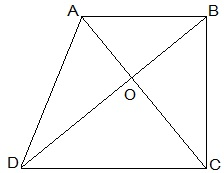△DAC and △DBC lie on the same base DC and between the same parallels AB and CD.
∴  ar(△DAC) = ar(△DBC)
⇒ ar(△DAC) − ar(△DOC) = ar(△DBC) − ar(△DOC)
ar(△AOD) = ar(△BOC)

11. In Fig. 9.27, ABCDE is a pentagon. A line through B parallel to AC meets DC produced at F.
Show that
(i) ar(ACB) = ar(ACF)
(ii) ar(AEDF) = ar(ABCDE)(i) △ACB and △ACF lie on the same base AC and between the same parallels AC and BF.
∴ ar(△ACB) = ar(△ ACF)

(ii) ar(△ACB) = ar(△ACF)
⇒ ar(△ACB) + ar(△ACDE) = ar(△ACF) + ar(△ACDE)
⇒ ar(ABCDE) = ar(△AEDF)

Page No: 164

12. A villager Itwaari has a plot of land of the shape of a quadrilateral. The Gram Panchayat of the village decided to take over some portion of his plot from one of the corners to construct a Health Centre. Itwaari agrees to the above proposal with the condition that he should be given equal amount of land in lieu of his land adjoining his plot so as to form a triangular plot. Explain how this proposal will be implemented.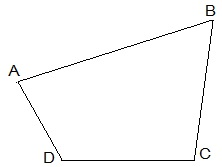Let ABCD be the plot of the land of the shape of a quadrilateral.Construction,
Diagonal BD is joined. AE is drawn parallel BD. BE is joined which intersected AD at O. △BCE is the shape of the original field and △AOB is the area for constructing health centre. Also, △DEO land joined to the plot.
To prove:
ar(△DEO) = ar(△AOB)
Proof:
△DEB and △DAB lie on the same base BD and between the same parallel lines BD and AE.
ar(△DEB) = ar(△DAB)
⇒ ar(△DEB) - ar△DOB) = ar(△DAB) - ar(△DOB)
⇒ ar(△DEO) = ar(△AOB)

13. ABCD is a trapezium with AB || DC. A line parallel to AC intersects AB at X and BC at Y. Prove that ar (ADX) = ar (ACY).
[Hint : Join CX.]Given,
ABCD is a trapezium with AB || DC.
XY || AC
Construction,
CX is joined.
To Prove,
Proof:
ar(△ADX) = ar(△AXC) --- (i) (On the same base AX and between the same parallels AB and CD)
also,
ar(△ AXC)=ar(△ ACY) --- (ii) (On the same base AC and between the same parallels XY and AC.)
From (i) and (ii),

14. In Fig.9.28, AP || BQ || CR. Prove that
ar(AQC) = ar(PBR).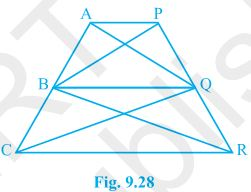Given,
AP || BQ || CR
To Prove,
ar(AQC) = ar(PBR)
Proof:
ar(△AQB) = ar(△PBQ) --- (i) (On the same base BQ and between the same parallels AP and BQ.)
also,
ar(△BQC) = ar(△BQR) --- (ii) (On the same base BQ and between the same parallels BQ and CR.)
ar(△AQB) + ar(△BQC) = ar(△PBQ) + ar(△BQR)
⇒ ar(△ AQC) = ar(△ PBR)

15. Diagonals AC and BD of a quadrilateral ABCD intersect at O in such a way that ar(AOD) = ar(BOC). Prove that ABCD is a trapezium.Given,
ar(△AOD) = ar(△BOC)
To Prove,
ABCD is a trapezium.
Proof:
ar(△AOD) = ar(△BOC)
⇒ ar(△AOD) + ar(△AOB) = ar(△BOC) + ar(△AOB)
Areas of △ADB and △ACB are equal. Therefore, they must lying between the same parallel lines.
Thus, AB ∥  CD
Therefore, ABCD is a trapezium.

16. In Fig.9.29, ar(DRC) = ar(DPC) and ar(BDP) = ar(ARC). Show that both the quadrilaterals ABCD and DCPR are trapeziums.Given,
ar(DRC) = ar(DPC) and ar(BDP) = ar(ARC)
To Prove,
ABCD and DCPR are trapeziums.
Proof:
ar(△BDP) = ar(△ARC)
⇒ ar(△BDP) - ar(△DPC) = ar(△DRC)
ar(△BDC) = ar(△ADC). Therefore, they must lying between the same parallel lines.
Thus, AB ∥ CD
Therefore, ABCD is a trapezium.
also,
ar(DRC) = ar(DPC). Therefore, they must lying between the same parallel lines.
Thus, DC ∥ PR
Therefore, DCPR is a trapezium.

## NCERT Solutions for Class 9 Maths Chapter 9 Areas of Parallelograms and Triangles

In the Chapter 9 Areas of Parallelograms and Triangles, we are focused on the relationship between the areas of these geometric figures under the condition when they lie on the same base and between the same parallels.

• Figures on the Same Base and Between the Same Parallels: Two figures are said to be on the same base and between the same parallels, if they have a common base (side) and the vertices (or the vertex) opposite to the common base of each figure lie on a line parallel to the base.

• Parallelograms on the same Base and Between the same Parallels: Parallelograms on the same base and between the same parallels are equal in area.

• Triangles on the same Base  and between the same Parallels: Two triangles on the same base (or equal bases) and between the same parallels are equal in area. Two triangles having the same base (or equal bases) and equal areas lie between the same parallels.

There are total 4 exercises in the Chapter 9 Class 9 Maths NCERT Solutions which are very important for examinations purpose. We have prepared exercisewise NCERT Solutions of every questions which can find from the given links.

NCERT Solutions prepared by Studyrankers subject matter experts will give you in depth insights of every question so you can always revise topics in less time.

### NCERT Solutions for Class 9 Maths Chapters:

 Chapter 1 Number Systems Chapter 2 Polynomials Chapter 3 Coordinate Geometry Chapter 4 Linear Equations in Two Variables Chapter 5 Introduction to Euclid’s Geometry Chapter 6 Lines and Angles Chapter 7 Triangles Chapter 8 Quadrilaterals Chapter 10 Circles Chapter 11 Constructions Chapter 12 Heron's Formula Chapter 13 Surface Areas And Volumes Chapter 14 Statistics Chapter 15 Probability

FAQ on Chapter 9 Areas of Parallelograms and Triangles

#### How can I download Chapter 9 Areas of Parallelograms and Triangles Class 9 NCERT Solutions PDF?

You can easily download Chapter 2 Polynomials NCERT Solutions just by visiting this page as it will help you in clearing your doubts just at a click. These NCERT Solutions are updated as per the latest marking pattern of CBSE.

#### How many exercises in Chapter 9 Areas of Parallelograms and Triangles?

There are total four exercises in the Chapter 9 Class 9 Maths in which the last one ins optional. We have provided accurate and also detailed every step through which one can always clear their doubts.

#### How can I understand the topics given in Chapter 9 Class 9 Maths?

NCERT Solutions is one of the best way through which one can understand all the topics given in the Chapter 9 Class 9 Maths. Through the help of these solutions, one will be able to solve supplementary books and exemplar questions as well.

#### What is Area of a parallelogram?

Area of a parallelogram = base × height.